## research papersJOURNAL OFAPPLIEDCRYSTALLOGRAPHY
ISSN: 1600-5767
Volume 49| Part 3| June 2016| Pages 1021-1028

## Identification of rogue datasets in serial crystallography1

aDepartment of Biology, University of Konstanz, Box 647, Konstanz, D-78457, Germany
*Correspondence e-mail: kay.diederichs@uni-konstanz.de

(Received 24 December 2015; accepted 1 April 2016; online 18 April 2016)

Advances in beamline optics, detectors and X-ray sources allow new techniques of crystallographic data collection. In serial crystallography, a large number of partial datasets from crystals of small volume are measured. Merging of datasets from different crystals in order to enhance data completeness and accuracy is only valid if the crystals are isomorphous, i.e. sufficiently similar in cell parameters, unit-cell contents and molecular structure. Identification and exclusion of non-isomorphous datasets is therefore indispensable and must be done by means of suitable indicators. To identify rogue datasets, the influence of each dataset on CC1/2 [Karplus & Diederichs (2012). Science, 336, 1030–1033], the correlation coefficient between pairs of intensities averaged in two randomly assigned subsets of observations, is evaluated. The presented method employs a precise calculation of CC1/2 that avoids the random assignment, and instead of using an overall CC1/2, an average over resolution shells is employed to obtain sensible results. The selection procedure was verified by measuring the correlation of observed (merged) intensities and intensities calculated from a model. It is found that inclusion and merging of non-isomorphous datasets may bias the refined model towards those datasets, and measures to reduce this effect are suggested.

### 1. Introduction

Several bottlenecks hamper structure determination of biological macromolecules. One practical problem is often the lack of suitably large crystals for collection of complete high-resolution data, as the diffraction signal for a given incident X-ray beam is proportional to the well ordered crystal volume illuminated by the beam, as given by Darwin's formula (Darwin, 1914, 1922; Blundell & Johnson, 1976). Smaller crystals require higher X-ray beam intensities to produce diffraction up to the same resolution as comparable crystals with larger volume, but because of radiation damage do not result in complete datasets.

This problem of incompleteness has been addressed by combining several partial datasets from multiple crystals in order to obtain a complete dataset averaged (merged') over all observations of every unique reflection. Merging of data from a few (2–20) crystals has been standard practice in crystallography (Kendrew et al., 1960; Dickerson et al., 1961), but recently this concept was extended to tens or even thousands of crystals with only a few reflections per dataset and termed serial crystallography (SX). When using extremely short pulses of X-rays, each of tens of femtoseconds in duration, generated by a free-electron laser (FEL) the method is referred to as serial femtosecond crystallography (SFX; Chapman et al., 2011). This also exploits the diffraction before destruction' approach, where single diffraction snapshots' are collected before radiation damage can occur. Serial crystallography performed at the synchrotron (SSX; Rossmann, 2014) is a more recent development based on data collection and processing methods established for single-crystal work, but enhanced by procedures for crystal identification by scanning crystallization plates using conventional optics or X-rays. This approach is also ideally suited for employing novel crystallization setups such as the lipidic cubic phase and in situ data collection at room or cryo temperature (Huang et al., 2015, 2016).

Although serial crystallography, especially SFX, can in principle solve or mitigate the problem of radiation damage and the lack of sufficiently large crystals, one potential obstacle is non-isomorphism of crystals. In order to merge different partial datasets into one complete dataset, one must ensure that all partial datasets are sufficiently isomorphous. Isomorphous crystals are structurally identical and correspond to the same atomic model such that datasets only differ by random error, whereas non-isomorphous crystals represent different structural features, at the level of cell parameters, composition (in terms of presence of molecular entities like ligands and solvent molecules) or molecular conformation. The variation between datasets thus also depends on the extent of non-isomorphism.

To group datasets on the basis of similarity, hierarchical clustering based on pairwise correlation coefficients was employed by Giordano et al. (2012). The basic idea here is that a low correlation coefficient indicates unrelatedness of datasets, which is interpreted as non-isomorphism. This method may also falsely reject datasets with a high level of random error, or in other words, weak datasets. Essentially, this would trade accuracy for precision.

Another method that can be employed is hierarchical clustering of datasets based on their cell parameters (Foadi et al., 2013). This method avoids the problem of false positive rejection of weak datasets, but on the other hand similarity of cell parameters is only a necessary but not a sufficient condition, and does not take similarity of diffraction into account. The criterion can therefore be considered as a rather weak filter.

Yet another approach combines the unit-cell changes, the intensity correlation coefficient and a relative anomalous correlation coefficient for clustering of datasets (Liu et al., 2013).

Following previous work (Karplus & Diederichs, 2012; Diederichs & Karplus, 2013), we chose the numerical value of CC1/2, an indicator for the precision of the data resulting from merging of the partial datasets, as an optimization target. We have shown earlier that CC1/2 limits – as seen from the properties of the derived quantity CC* – the ability to obtain good agreement between model and data (Karplus & Diederichs, 2012). Since the goal of structure analysis is to obtain a model consistent with the data, it appears logical to optimize CC1/2.

CC1/2 can be evaluated and optimized as a function of the datasets being merged, and we propose and study a procedure to identify non-isomorphous crystals from multi-crystal datasets based on their influence on CC1/2. Simulated data as well as experimental datasets from two projects, PepT and AlgE (Huang et al., 2015), were analysed to identify non-isomorphous datasets.

### 2. Methods

#### 2.1. Simulated data

Eleven complete datasets to 1.46 Å resolution were simulated with SIM_MX (Diederichs, 2009) using the atomic coordinates of cubic insulin [Protein Data Bank (PDB) code 2bn3 (Nanao et al., 2005); space group I213] and a flat bulk solvent (density 0.35 e A−3 and B = 50 Å2) for the calculation of structure factors. To simulate a specific case of non-isomorphism by numerical experiments, the unit-cell parameters were elongated by different amounts (0–1 Å). This results in a different sampling of the molecular transform and thus changes the intensities. Artificial Fcalc2 to be used as intensities were then calculated using PHENIX.FMODEL (Adams et al., 2002), and simulated raw datasets were calculated with SIM_MX and processed with XDS (Kabsch, 2010a,b). Different random seeds ensured that different (pseudo-) random errors for different datasets were calculated.

#### 2.2. Experimental data

Crystallization and data collection of the membrane protein peptide transporter PepT from Streptococcus thermophilus (483 residues) and AlgE, the alginate transporter from Pseudomonas aeruginosa (490 residues), were described previously (Huang et al., 2015). PepT and AlgE X-ray diffraction data consisting of small rotation wedges of different crystals, to 2.78 and 2.54 Å resolution, respectively, were collected at room temperature from crystals in meso and in situ at the PX II beamline of the Swiss Light Source (Villigen, Switzerland). Each rotation wedge was collected from a different crystal and is denoted as a (partial) dataset in the following. Data were processed with XDS and XSCALE (Kabsch, 2010a,b). For this work, not all datasets that were used at the time of PDB deposition were available; thus for PepT, we used 159, and for AlgE, we used all 266 datasets available, 175 of which were used in the work of Huang et al. (2015). Table 1summarizes these data.

 Table 1Crystallographic statistics of experimental datasets
PepT AlgE
PDB code 4xni 4xnk
Space group (20) C2221 (19) P212121
Unit-cell parameters (Å) a = 106.88, b = 106.88, c = 111.14 a = 48.01, b = 74.34, c = 184.69
Wavelength (Å) 0.979180 1.033000
No. of crystals 159 266
Resolution (Å) 50–2.78 50–2.54
Completeness (%) 97.6 84.7
Completeness highest resolution shell 67.6 (2.85–2.78) 7.6 (2.61–2.54)
Total No. of observations 905 207 151 228
No. of observations per crystal (min–max, mean) 2592–6040, 5693 59–507, 564
No. of unique reflections 16 485 18 684
Rmeas 0.973 0.565
CC1/2 0.992 0.926
I/σ(I)〉 4.25 2.74

#### 2.3. Calculation of CC1/2: the σ–τ method

For the calculations of CC1/2 the XSCALE output file (XSCALE.HKL) was used. Merged intensities of observations were weighted with their sigma values as assigned by the data processing programs, XDS and XSCALE.

As defined by Karplus & Diederichs (2012), CC1/2 can be calculated from the formula for Pearson's correlation coefficient: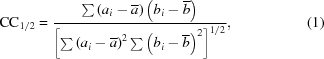where ai and bi are the intensities of unique reflections merged across the observations randomly assigned to subsets A and B, respectively, and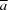and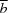are their averages.

For this work, we calculated CC1/2 in a different way, avoiding the random assignment to subsets. CC1/2 may be expressed as (Karplus & Diederichs, 2012, supplement)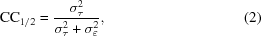with

τ = difference between true values of intensities and their average (thus τ has zero mean),

A,B = random errors in merged intensities of half-sized subsets (with zero mean) which are mutually independent and on average of equal magnitude,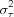= variance of τ,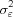= variance of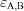.

Then, the full dataset merged intensity y (after subtraction of the average) is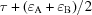and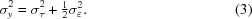We may thus substitute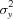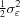forand obtain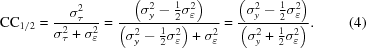This just requires calculation of, the variance of the average intensities across the unique reflections of a resolution shell, and, the average variance of the observations contributing to the merged intensities.

As shown above, this στ method' of CC1/2 calculation is mathematically equivalent to the calculation of a Pearson correlation coefficient for the special case of two sets of values (intensities) that randomly deviate from their common true' values. Since it avoids the random assignment into half-datasets, it is not influenced by any specific random number sequence and thus yields more consistent values, as further discussed below (§4.1).

The average intensity of macromolecular diffraction data diminishes with increasing resolution. If all data, from low to high resolution, are used for calculation of an overall CC1/2, the resulting correlation coefficient is dominated by the strong low-resolution terms and therefore biased towards large positive values; the overall CC1/2 is thus almost meaningless (Karplus & Diederichs, 2015). More meaningful CC1/2 values are obtained when dividing the data into (usually ten or more) resolution shells, since in each resolution shell the average intensity can be considered constant. To obtain a single value, we average the CC1/2 values obtained in all resolution shells, while weighting with the number of contributing reflections.

#### 2.4. The ΔCC1/2 method

Since our goal is to maximize CC1/2 by excluding datasets, we used the following simple algorithm that avoids any combinatorial explosion of possibilities. We define as CC1/2_overall the value of the average CC1/2 when all datasets are included for calculation. Furthermore, we denote as CC1/2_i the value of CC1/2 when all datasets are included except for one dataset i, which is omitted from calculations. CC1/2_i is calculated for every dataset i. We define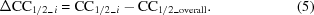If ΔCC1/2_i > 0 (< 0), this dataset is improving (impairing) the overall CC1/2. After rejection of the dataset belonging to the worst negative ΔCC1/2_i, all remaining datasets are processed by XSCALE again, because any dataset influences all scale factors. The newly scaled output file can be used to identify another potential non-isomorphous dataset, and this may be iterated until no further significant improvement is obtained; usually, two or three iterations are sufficient.

#### 2.5. Validation of isomorphous dataset selection

We devised a strategy to assess an actual improvement of the data by comparison with the squared Fcalc moduli obtained from a structural model. To this end, deposited PDB models (4xnj for PepT, 4xnk for AlgE; Huang et al., 2015) were processed with PHENIX.FMODEL to produce Fcalc2 reference data, to be used for comparison with Iobs. We define CCFOC_overall as the correlation coefficient of observed and calculated intensities when all datasets are included. Moreover, we define CCFOC_i as the correlation coefficient with all datasets included except for one dataset i, which is omitted from calculations. The difference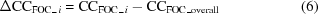then gives the improvement or impairment the dataset i causes in the overall correlation of data and model. ΔCCFOC_i < 0 indicates an impairment in the similarity of data and model; ΔCCFOC_i > 0 indicates an improvement. We chose to compare ΔCCFOC_i and ΔCC1/2_i although it would be more appropriate to use CC* (Karplus & Diederichs, 2012) instead of CC1/2, or in other words, to compare ΔCCFOC_i and a quantity defined analogously to ΔCC1/2_i, ΔCC*i = CC*i CC*overall. However, since CC* depends monotonically on CC1/2, any qualitative finding obtained in a comparison of ΔCCFOC_i and ΔCC1/2_i would be the same as for a ΔCCFOC_i and ΔCC*i comparison.

For some calculations, random shifts of atom coordinates of the original PDB file were introduced by MOLEMAN2 (Kleywegt, 1995).

### 3. Results

#### 3.1. Characteristics of ΔCC1/2 for the simulated data

Eleven complete datasets with different changes in the unit-cell parameters were used to simulate a realistic case where most of the datasets are isomorphous relative to each other but some are (non-isomorphous) outliers.

The ΔCC1/2 method was applied to the simulated datasets. In general, increasing changes in unit-cell parameters are associated with decreasing ΔCC1/2_i, as expected (Table 2). The largest change in unit-cell parameters (1.0 Å) shows the lowest ΔCC1/2_i, which is thus correctly identifying non-isomorphism. ΔCC1/2_i shows highly positive values for those datasets where no or only slight changes were introduced (0.0–0.2 Å). ΔCC1/2_i does not increase linearly; rather, it drops dramatically from 0.2 to 0.4 Å. On the basis of ΔCC1/2_i, the most isomorphous dataset is the one with a 0.2 Å cell parameter change; this appears to be a sensible result since its intensities are the most closely related to those of all other datasets.

 Table 2ΔCC1/2_i of synthetic datasets with elongated unit-cell parameters
Change of unit-cell parameters (Å) ΔCC1/2_i
+1.0 −0.518
+0.8 −0.313
+0.6 −0.271
+0.4 −0.262
+0.2 +0.873
+0.1 (2 datasets) +0.785, +0.785
0.0 (4 datasets) +0.710, +0.706, +0.698, +0.684

The numerical values of ΔCC1/2_i change to a much greater extent (−0.5 to 0.8) than in the experimental case. This is because the impact of one complete dataset (out of 11) is high in comparison to a single small rotation wedge SSX dataset (out of hundreds). Moreover, the artificially induced gap between nearly perfect isomorphous datasets (cell changes of 0.0–0.4 Å, i.e. close to the average of all cell changes) and very few severely non-isomorphous datasets (cell changes of 0.6–1.0 Å) enforces this effect.

#### 3.2. PepT: model unbiased by non-isomorphous dataset

For PepT, 159 datasets were analysed, as seen in Fig. 1, where a histogram of ΔCC1/2_i is shown. The histogram is dominated by a Gaussian-shaped central part slightly above ΔCC1/2 = 0, with standard deviation σ = 1.68 × 10−4. Datasets with a ΔCC1/2_i of around zero do not significantly change the overall CC1/2; however, their inclusion is necessary for increased completeness and multiplicity. One dataset has a significantly (−28.8σ) negative ΔCC1/2_i and is thus identified as non-isomorphous. Some datasets have, at 31.8σ and 76.2σ, a highly positive ΔCC1/2_i; they significantly decrease CC1/2_overall if rejected and appear to be particularly valuable.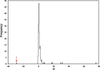Figure 1 Histogram of ΔCC1/2_i values for PepT. The −28.8σ unit outlier is indicated with an arrow.

Comparing, in terms of raw data appearance and processing logfiles and statistics reported by XDS or XSCALE, positive or negative outlier datasets with datasets from the central part of the histogram showed no striking peculiarities for any of the analysed criteria such as number of reflections or similar. In particular, negative ΔCC1/2_i was not predictable from unit-cell parameters, spot shape or other data processing statistics of single datasets. Furthermore, the negative outlier had not been identified by the ISa-based (Diederichs, 2009) rejection of datasets performed by Huang et al. (2015). However, we note that important experimental variables, like crystal volume, are not recorded during the experiments.

Fig. 2shows a plot of ΔCCFOC_i against ΔCC1/2_i for the datasets of this project. If our procedure for identifying non-isomorphism is meaningful, we expect an improvement of the correlation between model Fcalc2 and merged Iobs for those datasets that increase CC1/2, and a decrease of correlation for the non-isomorphous ones. As could be expected from the histogram of Fig.1, most of the data sets cause little change of ΔCCFOC and ΔCC1/2; they cluster in the middle of the diagram. The dataset identified as the most non-isomorphous one indeed shows the worst correlation of experimental data and model; CCFOC is significantly improved when rejecting this specific dataset. Conversely, some of the datasets show a clear improvement of CCFOC and CC1/2.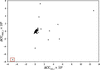Figure 2 Plot of ΔCCFOC_i against ΔCC1/2_i for PepT. The −28.8 8σ unit outlier (ΔCC1/2_i ≃ −4.8 × 10−4) is boxed.

The validation appears to work satisfactorily despite the fact that the 4xnj model derived from cryo data and used here for validation is itself not isomorphous with the data, since the cell parameters of the cryo and the room-temperature crystals differ in a and b by about 4%.

#### 3.3. AlgE: model biased by non-isomorphous dataset

AlgE displays a similar histogram (σ = 7.22 × 10−4) of ΔCC1/2_i values (Fig. 3) as PepT. The worst non-isomorphous dataset is, at −14.8σ units, an obvious outlier of the ΔCC1/2_i distribution. Similarly, positive outliers exist at 10.1σ and 11.5σ units. As for PepT, we did not observe in the raw data or in the processing and scaling statistics any particular properties of positive or negative outliers. Again, the strongest negative outlier had not been identified by the ISa-based rejection of datasets performed by Huang et al. (2015).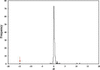Figure 3 Histogram of ΔCC1/2_i values for AlgE. The −14.8σ unit outlier is indicated with an arrow.

In contrast to Fig. 2, the plot of ΔCCFOC_i against ΔCC1/2_i for AlgE shows an unexpected behaviour (Fig. 4). As expected, most of the datasets cluster at ΔCC1/2 and ΔCCFOC values around zero, but the dataset identified by ΔCC1/2_i as the most non-isomorphous dataset is surprisingly showing the best ΔCCFOC_i. Conversely, the best datasets as judged from ΔCC1/2_i exhibit negative ΔCCFOC_i.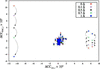Figure 4 Plot of ΔCCFOC_i against ΔCC1/2_i for AlgE. Different colours and marker symbols refer to the different random shifts of the atom coordinates. Arrows indicate the change of ΔCCFOC_i upon increasing the magnitude of random shifts for the three most significant outliers of the Gaussian distribution of Fig. 3.

After some investigation, we attributed these observations to our choice of PDB models used for validation. In the case of PepT, we had chosen the model based on an independent single-crystal cryo dataset (4xnj), whereas in the case of AlgE, we had chosen 4xnk which had been refined against those datasets we were now trying to characterize. In the case of AlgE, refinement of the model 4xnk against the SSX data had led to a bias in the sense that the model partly fits the systematic effects contributing to the non-isomorphism of the worst dataset. This bias additionally leads to a reduced ΔCCFOC_i since the model is biased into a state more distant from those of datasets with positive ΔCC1/2_i.

We therefore performed an additional experiment: By applying random shifts of magnitude up to 1.0 Å to the 4xnk coordinates, we attempted to shake' the model out of its local minimum. Recalculating ΔCCFOC_i for increasing shifts, we progressively observed the expected behaviour of a positive correlation between ΔCC1/2_i and ΔCCFOC_i, as emphasized by arrows in Fig. 4. This is most pronounced for the dataset with largest negative ΔCC1/2_i, which is seen to have strongly negative ΔCCFOC_i when the model is shaken most. However, the datasets with most positive ΔCC1/2_i did not fully reach high values of ΔCCFOC_i, presumably because, for the highest shifts, the model is strongly degraded so that it cannot fit the data well.

Ideally, validation is done with a model that is independent of the data. Another experiment was therefore performed with the 4xnl coordinates derived from a dataset collected at cryo temperature. In this case, no bias is present, and indeed there is a positive correlation between ΔCC1/2_i and ΔCCFOC_i (data not shown), similar to Fig. 2for PepT, as expected.

### 4. Discussion

#### 4.1. Calculation of CC1/2

We have shown above that CC1/2 can be calculated with the στ method, without invoking random selections of observations. The approach to the calculation of CC1/2 has a number of advantages compared with the random-selection method using the formula for Pearson's correlation coefficient:

(a) It avoids the numerical spread of results associated with different seeds of the random split assignment and is therefore more accurate.

(b) It treats odd numbers of reflections consistently, which otherwise lead to unequal numbers in the two half-datasets, which again leads to more accurate results.

(c) It treats the sigma weighting of merged intensities more consistently. The original derivation of properties of CC1/2 (Karplus & Diederichs, 2012, supplement) does not take weighting of intensities into account, whereas our formulation in §2.3naturally accommodates weighted intensities.

(d) The higher precision of CC1/2 values allows us to calculate precise ΔCC1/2 values that would vanish in the noise incurred by random assignments.

(e) The calculation of the anomalous CC1/2 (CC1/2_ano) can be done analogously. For CC1/2_ano, the formula suggests an answer to a question that has puzzled several crystallographers and was discussed on the CCP4 bulletin board (CCP4 Bulletin Board, 2015): why do we sometimes see negative (mostly anomalous) CC1/2 values in high-resolution shells? The formula tells us immediately that this symptom indicates that the average variance of the observations is higher than the variance of the averaged intensities in those particular resolution shells. Obviously, this is consistent with the given situation in which practically no anomalous signal but the usual measurement error is present.

#### 4.2. Choice of target function and rejection criterion

There exist two types of errors in crystallographic intensity data: random and systematic. If only random errors are present in the observed intensities Ii, no datasets should be discarded, no matter how weak they are, since they improve the merged intensities Imerged if the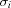are derived from counting statistics. This situation defines `isomorphism of datasets', in which the following hold:

(i) The relations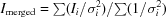and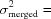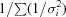hold strictly, and therefore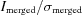grows monotonically if more data are merged.

(ii)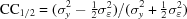(as defined in §2) also grows monotonically since the mean error decreases if more data are merged. The value of CC* = [ 2 CC1/2 / (1 + CC1/2 )]1/2, itself monotonically depending on CC1/2, then is an accurate indicator for the correlation of the merged data and the (unknown) true data (Karplus & Diederichs, 2012).

However, if systematic errors exist – which is unfortunately always the case, to some extent – these are by definition not independent. The above relations for Imerged,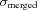and CC1/2 then tell us the precision, but not the accuracy, of the merged intensities.

Theratio still increases in the presence of systematic errors, since the denominator of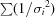grows with every observation merged.is therefore not suitable for identifying systematic error. CC1/2, on the other hand, diminishes if data with a sufficient amount of systematic error are merged, since it depends on the agreement of the observed intensity values. Non-isomorphism between datasets is a special case of systematic errors which affect all reflections in a dataset in a way that may in principle be different for every dataset. For simplicity, however, we may assume that most datasets do not differ significantly in systematic ways. This assumption is valid if the crystals are grown from the same protein preparation under the same conditions, the crystals are mounted, measured and processed in the same (or a closely similar) way, and indexing ambiguities (if applicable) have been resolved (Brehm & Diederichs, 2014).

A single rogue (outlier) dataset then has a small influence on the merged data, but if even the small part of the total dataset that it influences leads to a significant decrease of CC1/2, this may be considered a strong hint towards non-isomorphism of this particular dataset, and it appears justified to discard it. Its exclusion should reduce the noise in electron density maps and improve the agreement between merged data and the refined model.

A small degree of non-isomorphism in a dataset may still allow a slight increase or lead to an insignificant decrease of CC1/2, such that this dataset cannot be identified with the ΔCC1/2 method. If a large number of such datasets are merged, this will lead to a degradation of the merged intensities, because they introduce into the merged data a mixture of signals corresponding to molecular conformations or states distant from the majority one. Refinement of a single model against such merged data will ultimately also result in elevated Rwork/Rfree and noise in electron density maps. This means that many slightly non-isomorphous datasets may result in a slight increase of CC1/2 while nevertheless decreasing the suitability of the data for refinement.

An increase of CC1/2 is thus a necessary but, because of this caveat, not strictly a sufficient condition for improvement of data by merging. In principle, the ΔCC1/2 method shares this restriction with the BLEND method (Foadi et al., 2013), which uses a large cell parameter deviation as rejection criterion. However, since it uses the experimental intensity data, the ΔCC1/2 method directly targets the desired property of optimizing the merged intensity data, and is successful in doing so as seen when being validated. Compared to the pairwise-correlation method of Giordano et al. (2012), which interprets low correlation as meaning low non-isomorphism, we argue that our method avoids the erroneous rejection of weak datasets, at least in situations where the majority of datasets are isomorphous and a mixture of strong and weak datasets exists.

#### 4.3. Non-isomorphism in simulated and experimental data

If datasets are artificially modified such that non-isomorphism is introduced by increasing amounts of unit-cell inflation, a direct relation between ΔCC1/2_i and the amount of unit-cell change is found (Table 2). We find that changes in the unit cell from 0.4 Å can be considered as non-isomorphous for this combination of datasets. This does not mean that non-isomorphism caused by unit-cell changes is in general not detectable below 0.4 Å; in fact the threshold is dependent on the resolution of the data and the specific combination of datasets, which is why we propose an iterative usage of the method.

The most isomorphous dataset is the one with the average of all unit-cell dimensions, which appears to confirm the method of Foadi et al. (2013). The latter method would not have been of much help for the PepT and AlgE projects, however, because their partial datasets have poorly determined unit-cell parameters and therefore our a posteriori analysis could not reveal any particular unit-cell-related deviations or properties of non-isomorphous datasets.

For the latter projects, identification of non-isomorphous datasets was straightforward with the ΔCC1/2 method. Owing to our precise method for CC1/2 calculation, outliers may yield high significance levels, and we expect this to hold also for a larger number of datasets.

Unfortunately, from a theoretical point of view it remains unclear which properties the outlier datasets have such that they strongly influence the merged data; further work in this area is underway.

#### 4.4. Pitfalls of validation

One way of validating the identification of non-isomorphous datasets would be to refine a model against merged data with and without the dataset in question and to compare Rwork/Rfree of the two refinements. However, trials to do so convinced us that the small number of free-set reflections present in each partial dataset lead to inconclusive results as Rfree showed large variations.

Likewise, direct comparison of squared structure factors from the model with intensities of partial datasets did not lead to conclusive results, since weak datasets displayed low correlations.

We therefore compared, without refinement, ΔCCFOC_i, the change in correlation coefficient between observed structure factors and structure factors calculated from their PDB models, with ΔCC1/2_i. In the case of PepT, we found that those datasets which were identified as non-isomorphous according to ΔCC1/2_i also reduce the correlation of the merged data with the model, and thus we confirmed our decision based on ΔCC1/2_i. However, the AlgE datasets displayed the opposite effect, which puzzled us until we realized that the model we were basing the comparison upon had – in contrast to the PepT model we used – originally been refined against the very data we were comparing it with. In other words, the model had been influenced by all datasets, including non-isomorphous ones, and was therefore biased: exclusion of non-isomorphous data from the merged data resulted in an increase of ΔCCFOC_i. The remedy we found and employed was to add large random shifts with zero mean to the coordinates of the model, thus reducing the bias by forcing the model out of its biased local energy minimum, at the expense of an overall degraded model with little discriminatory power.

One way of avoiding the bias problem would be to perform refinements of a mildly shaken model and leave out each dataset in turn, to arrive at more realistic unbiased ΔCCFOC_i values. However, this would be a project on its own and was considered outside the scope of this work.

#### 4.5. Summary

Our findings demonstrate that non-isomorphous datasets can be identified with an algorithm which uses the numerical value of the average CC1/2 across all resolution shells as an optimization criterion. This not only works well with artificial data simulating cell parameter variation but is also demonstrated and validated with experimental data.

Although CC1/2 was devised as a precision indicator for the merged data, it can also serve as a proxy for the quality of the model that can be derived from the data, since CC1/2 constitutes the link between data and model quality (Karplus & Diederichs, 2012). This role as proxy is compromised if model bias plays a role. However, contrary to classical model bias where the phases and therefore the electron density map are influenced by the very model that is the goal of structure solution, here we experience a different kind of bias: a dataset influences the model to such an extent that the correlation of model and data always diminishes if that dataset is removed from the merged data (and is strong enough). This leads to the insight that each and every dataset noticeably influences the model, and consequently the model will have to account for all possible constituents and conformations present in the data.

However, systematic differences between crystals cannot properly be modelled in refinement since in serial crystallography the averaging of datasets is incoherently done on intensities, rather than on structure factors as would happen if different conformations occur in the coherently illuminated volume of a single crystal. Since a refined model, with its coherently diffracting constituents, cannot fully approximate a sum of intensities (i.e. squared amplitudes) with a (squared) sum of amplitudes, an elevated level of Rwork/Rfree and noise in electron density maps would result if non-isomorphism is not detected and the worst datasets are not excluded.

In our trials with experimental data measured at a synchrotron, the outcome was the rejection of only a single (as for PepT and AlgE) or a few rogue datasets. However, this rather attests to the consistent quality of the data obtained in SSX. Finally, we note that our procedure is equally applicable to datasets obtained from SFX. Rejection rates may be higher in the latter method since its technology is less mature than that of SSX and the absolute numbers of datasets are high. We therefore expect that the ΔCC1/2 method will be useful in SFX.

### Footnotes

1This article will form part of a virtual special issue of the journal on free-electron laser software.

### Acknowledgements

We are greatly indebted to Martin Caffrey and his group (Trinity College, Dublin, Ireland), as well as to Meitian Wang and his group (Paul-Scherrer-Institute, Villigen, Switzerland), for allowing us to use the PepT and AlgE datasets. The authors gratefully acknowledge funding and support by the Konstanz Research School Chemical Biology.

### References

This is an open-access article distributed under the terms of the Creative Commons Attribution (CC-BY) Licence, which permits unrestricted use, distribution, and reproduction in any medium, provided the original authors and source are cited.JOURNAL OFAPPLIEDCRYSTALLOGRAPHY
ISSN: 1600-5767
Volume 49| Part 3| June 2016| Pages 1021-1028